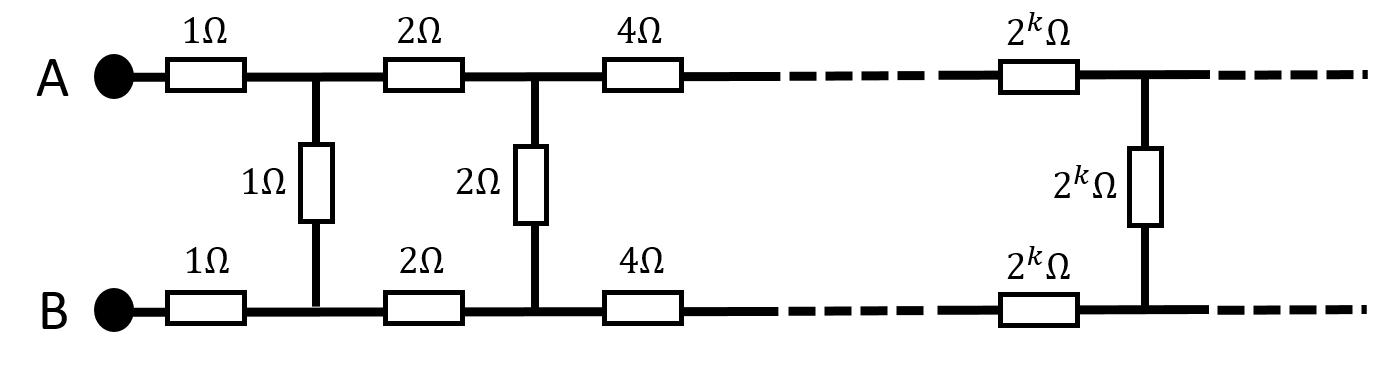# Infinitely Infinite ResistorsFind the resistance between the points $A$ and $B$ of an infinite circuit shown. The resistance of the resistors in each loop is twice those of the previous loop on its left.

Given that the resistance can be written as:

$\frac { a+\sqrt { b } }{ c }$

Find $a+b+c$

SJPO SPECIAL ROUND 2010

This question is part of my set SJPO Practice Questions

×